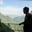Related Tags

python
pandas
dataframe
truediv()
communitycreator

# The DataFrame.truediv() method in Python pandasAKASH BAJWA

### The pandas library and DataFrame

pandas is a data analysis software package in Python for manipulating data. This library contains the class DataFrame.

A pandas DataFrame is a 2D data structure organized in rows and columns in a tabular format. It allows us to store tabular data and perform various operations on data using methods. The three main components of pandas DataFrame are:

• Principal data — the data on which the methods are applied.
• Rows.
• Columns.

### The DataFrame.truediv() method

Here, truediv() means true division, which means that the result will be float. The truediv() method will perform floating division on all elements of the DataFrame so that the resulting elements will be float even if they would otherwise be expected to be int.

### Syntax

The below line demonstrates the syntax of truediv() method.

DataFrame.truediv(other, axis = 'columns', level = None, fill_value = None)

### Parameters

The truediv() method takes one required parameter, while the other parameters are optional.

• other: This is the divisor. It can be a list-like object or a data structure with a single or multiple elements. This is a required parameter.
• axis: This determines the axis on which truediv() will operate. If set to 0 or 'index', it will operate on the index. When set to 1 or 'columns', it will operate on columns. This is an optional parameter.
• level: This determines the level in the supplied MultiIndex on which truediv() will match index values. This is an optional parameter
• fill_value: This specifies the value which will replace NaN values in the result. This can be a float value or None.

### Return value

The truediv() method will return a DataFrame with the intended results. All columns that truediv() operated on will contain float values.

### Example one

In our first example, we will use truediv() on a DataFrame with a scalar passed in the other argument.

# importing pandas as pd
import pandas as pd
# Creating a dataframe with three observations
df= pd.DataFrame({"S":[360,50,10],
"T":[50,0,30],
"U":[70,70,0]})
# Print the dataframe
print(df, "\n")
#dividing each and every value by 4
print(df.truediv(other = 4))
Hit run to see the results! Try changing the "other" input argument and observe the results.

### Explanation

• Line 2: We import the pandas library as pd.
• Lines 4–6: We create a DataFrame df with columns S, T, and U.
• Line 8: We print df.
• Line 10: We use truediv() on df with 4 as other.

We find the following in the output:

• All elements in the resulting DataFrame have been divided by 4.
• All elements in the resulting DataFrame are float.

### Example two

In our second example, we will divide a DataFrame with a Python list passed in the other argument. We will also set axis to 'columns'.

Note: 'columns' is the default value for axis.

# importing pandas as pd
import pandas as pd
# Creating a dataframe with three observations
df= pd.DataFrame({"S":[360,50,10],
"T":[50,0,30],
"U":[70,70,0]})
# Print the dataframe
print(df, "\n")
#dividing each and every value by 4
print(df.truediv(other = [20, 10, 50], axis='columns'))
Hit run to see the results! Try changing the "other" input argument and observe the results.

### Explanation

• Line 2: We import the pandas library as pd.
• Lines 4–6: We create a DataFrame df with columns S, T, and U.
• Line 8: We print df.
• Line 10: We use truediv() on df where other is a Python list with elements [20, 10, 50], and print the result.

We find the following in the output:

• Elements of the DataFrame df have been divided so that for every row, the first element has been divided with the first element of the list other, the second element has been divided with the second element of the list other, and so on.
• All elements in the resulting DataFrame are float.

### Example three

In our third example, we will divide a DataFrame with a pandas series passed in the other argument. We will also set axis to 'index'.

Note: When using truediv() with axis set to 'index', ensure that other has the same number of indexes as df.

# importing pandas as pd
import pandas as pd
# Creating a dataframe with three observations
df= pd.DataFrame({"A":[100,50,10],
"B":[50,20,30],
"C":[70,70,25]})
# Print the dataframe
print(df, "\n")
# subtracting with series type data
print(df.truediv(pd.Series([5, 10, 2], index=[0,1,2]), axis='index'))
Hit run to see the results! Try changing the "other" input argument and observe the results.

#### Explanation

• Line 2: We import the pandas library as pd.
• Lines 4–6: We create a DataFrame df with columns A, B, and C.
• Line 8: We print df.
• Line 10: We use truediv() on df where other is a pandas series with elements [5, 10, 2], and print the result.

We find the following in the output:

• Elements of the DataFrame df have been divided so that for every column, the first element has been divided with the first element of the series other, the second element has been divided with the second element of the series other, and so on.
• All elements in the resulting DataFrame are float.

RELATED TAGS

python
pandas
dataframe
truediv()
communitycreator

CONTRIBUTORAKASH BAJWA
RELATED COURSES

View all Courses

Keep Exploring

Learn in-demand tech skills in half the time